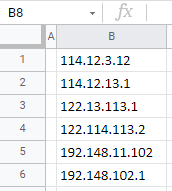This tutorial demonstrates how to sort IP addresses in Excel and Google Sheets.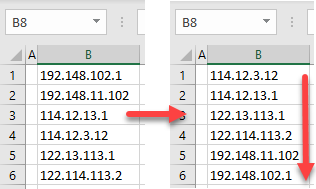IP addresses consist of four numbers (with a maximum length of three digits each) separated by points (periods). Excel can’t sort data in this format correctly. You first have to add leading zeros where needed to make all four numbers three characters in length. Say you have the list of IP addresses below.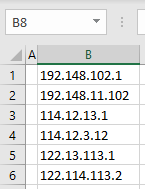To sort them correctly, follow these steps:

1. In cell C2, enter this formula that will add leading zeros to each number part of IP addresses:
``=TEXT(LEFT(B1,FIND(".",B1,1)-1),"000")&"."&TEXT(MID(B1,FIND(".",B1,1)+1,FIND(".",B1,FIND(".",B1,1)+1)-FIND(".",B1,1)-1),"000")& "."&TEXT(MID(B1,FIND(".",B1,FIND(".",B1,1)+1)+1,FIND(".",B1,FIND(".",B1,FIND(".",B1,1)+1)+1)-FIND(".",B1,FIND(".",B1,1)+1)-1),"000")&"."&TEXT(RIGHT(B1,LEN(B1)-FIND(".",B1,FIND(".",B1,FIND(".",B1,1)+1)+1)),"000")``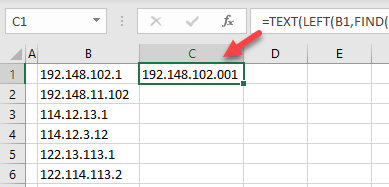1. Drag the formula down to the last populated row (6).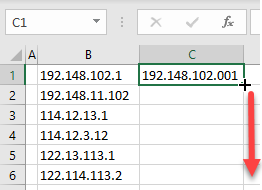1. Now all IP addresses numbers have any leading zeros necessary. Before sorting, copy and paste as values. Select the range with formulas (C1:C6), right-click the selected area, and choose Copy (or use the keyboard shortcut CTRL + C).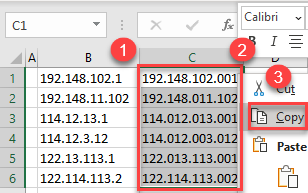1. Right-click the first cell in the next column (D1), and choose the Paste Values icon (or use the Paste Values shortcut).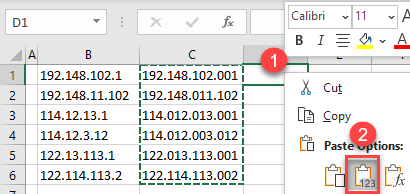1. Delete the column with formulas and sort the formatted data. Click somewhere in the formatted data range (Column C), and in the Ribbon, go to Home > Sort & Filter > Sort A to Z.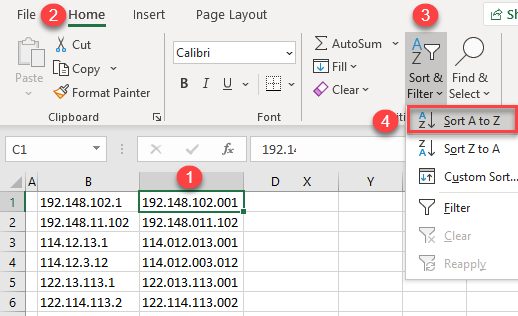As a result, IP addresses in Column B are sorted properly, and you can delete the helper column (C).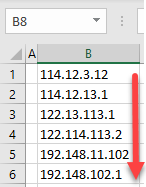### How Does the Formula Work?

The complex formula from Step 1 uses the TEXT, LEFT, MID, RIGHT, and FIND Functions to add leading zeros so each of the four number has a length of three.

• The FIND Function finds points in the IP address.
• The LEFT, MID, and RIGHT Functions extract each number.
• Finally, the TEXT Function formats each number to have a length of three numbers (“000”). This means that a number has one or two digits, two or one leading zeros are added, respectively). The ampersand (&) is used to join all numbers separated by points.

Using the same formula from Step 1 above, you can also sort IP addresses in Google Sheets.

1. In cell C2, enter the formula and drag it to the last populated row (6).
``=TEXT(LEFT(B1,FIND(".",B1,1)-1),"000")&"."&TEXT(MID(B1,FIND(".",B1,1)+1,FIND(".",B1,FIND(".",B1,1)+1)-FIND(".",B1,1)-1),"000")& "."&TEXT(MID(B1,FIND(".",B1,FIND(".",B1,1)+1)+1,FIND(".",B1,FIND(".",B1,FIND(".",B1,1)+1)+1)-FIND(".",B1,FIND(".",B1,1)+1)-1),"000")&"."&TEXT(RIGHT(B1,LEN(B1)-FIND(".",B1,FIND(".",B1,FIND(".",B1,1)+1)+1)),"000")``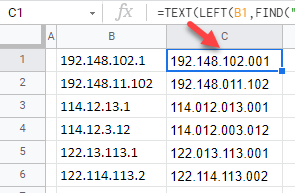1. Now all IP addresses numbers have leading zeros and a length of three. Before sorting, copy and paste as values. Select the range with formulas (C1:C6), right-click the selected are, and choose Copy (or use CTRL + C).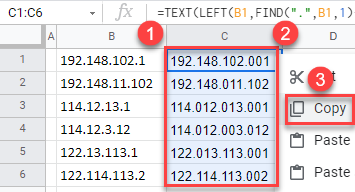1. Right-click the first cell in the next column (D1), click Paste special, and choose Values only (or use the CTRL + SHIFT + V shortcut).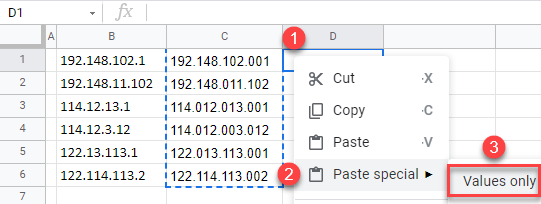1. Delete the column with formulas and sort the formatted data. Click somewhere in the formatted data range (Column C), and in the Menu, go to Data > Sort sheet > Sort sheet by column C (A to Z).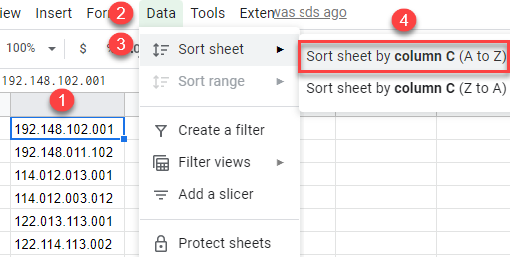As a result, IP addresses in Column B are sorted properly, and you can delete the helper column (C).